### Home > CALC > Chapter 10 > Lesson 10.2.2 > Problem10-107

10-107.
1. Evaluate the following limits. Homework Help ✎

1.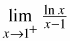2.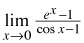3.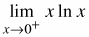4.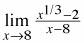5.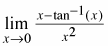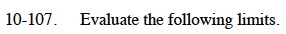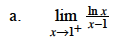Use one iteration of l'Hôpital's Rule.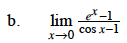Use one iteration of l'Hôpital's Rule.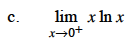Rewrite this as:

$\lim_{x\to 0^+}\frac{\ln(x)}{1/x}$

Then use l'Hôpital's Rule.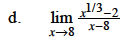Factor the denominator as a difference of cubes:
x3a3 = (xa)(x2 + axa2)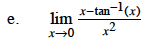Use two iterations of l'Hôpital's Rule.